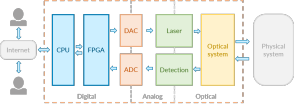# Build a Doppler lidar velocimeter

2015-11-15

We are excited to share our first Doppler lidar velocimeter built with our Laser Board for the Red Pitaya.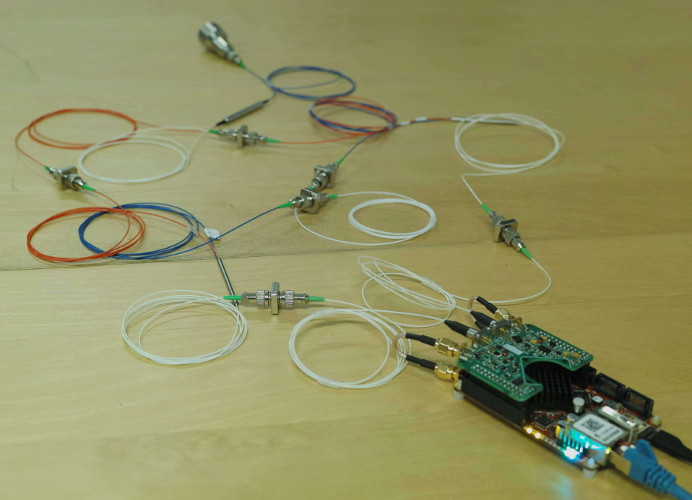Let's start with a quick explanation of the Doppler effect. We often experience it when hearing the siren of a moving vehicle: the tone gets higher when the vehicle is approaching.

The frequency of the acoustic wave emitted by the siren is affected by the movement of the vehicle. This effect is not only true for sounds but also for optical waves: when a laser beam with frequency $f_{0}$ is reflected off a moving target with velocity $v$, its frequency is shifted of a quantity

$$\Delta f = \frac{2v}{c} f_{0},$$

where $c$ is the speed of light. The factor 2 occurs because the light is not emitted by the target but reflected off it. Our laser emits at a frequency of 193.5 THz (i.e. a wavelength of 1550 nm). A target moving at 1 m/s would shift the laser frequency by 1.29 MHz.

## Measuring frequency shifts

Photodetectors are too slow to follow optical frequencies. Fortunately, the Doppler displacement $\Delta f$ of several MHz is low enough to be caught on a photodiode. To produce a modulation at the Doppler frequency, we use a beat-note: when two waves with frequencies $f_{1} = f_{0}$ and $f_{2} = f_{0} + \Delta f$ overlap, they beat at a frequency $f_{2} - f_{1} = \Delta f$.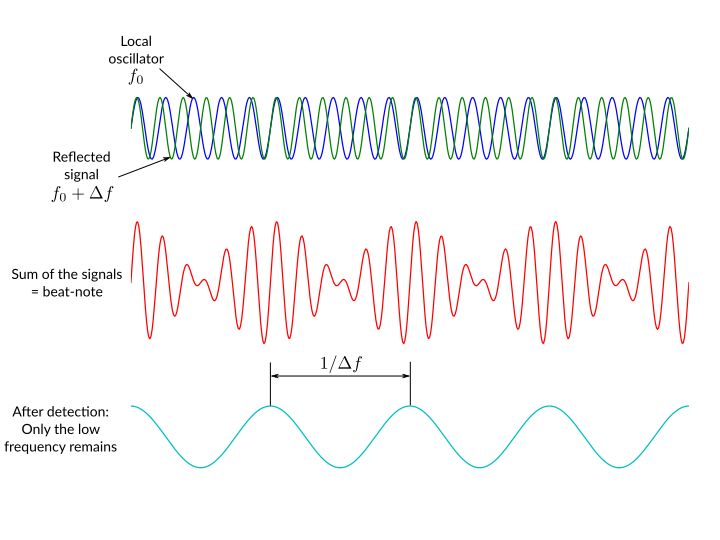## Lidar setup

The laser is split into two beams. One beam (probe) is sent to the target . The second one (local oscillator) acts as a frequency reference $f_{0}$. The probing beam exits the collimator, reflects on the target and is collected by the collimator. The optical circulator then redirects the signal to a photodiode. The signal is mixed with the local oscillator to produce the beat-note on the photodiode.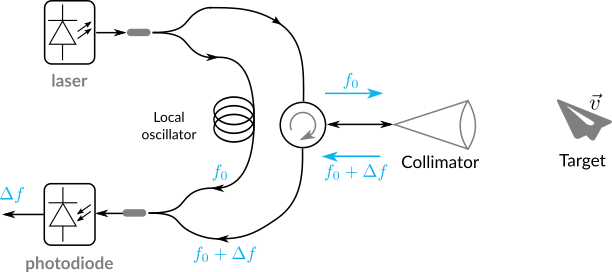The Doppler frequency appears as a peak in the signal spectrum. Thanks to the on-board FPGA, spectrums are computed in real-time and averaged on-the-fly, which dramatically improves signal-to-noise ratio. We measured the velocity of a custom usb fan.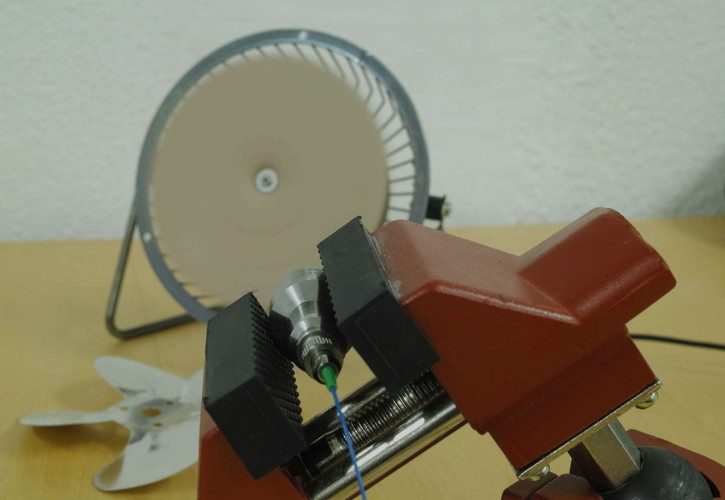Here is what we observe on the graphical interface (source code). The fan velocity varies over the laser beam, resulting in a broadened peak. The peak has a frequency of about 4.5 MHz, which corresponds to a speed of about 7 m/s.## Also tagged instrumentation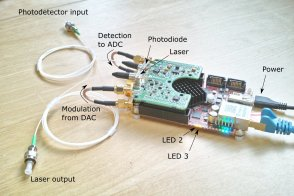### Control the laser board with Python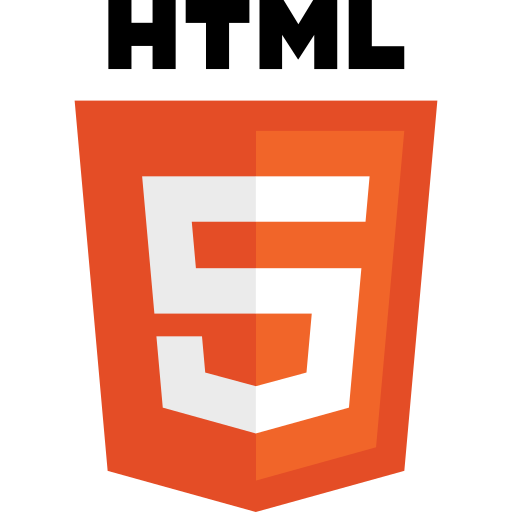### Web based interfaces for instrument control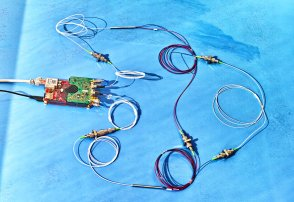### A simple coherent laser sensor